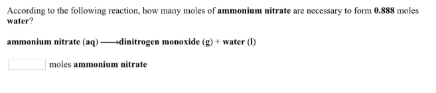# Problem: According to the following reaction, how many moles of ammonium nitrate are necessary to form 0.888 moles water? ammonium nitrate (aq) → dinitrogen monoxide (g) + water (1) moles ammonium nitrate

###### FREE Expert Solution
88% (364 ratings)###### Problem Details

According to the following reaction, how many moles of ammonium nitrate are necessary to form 0.888 moles water?

ammonium nitrate (aq) → dinitrogen monoxide (g) + water (1) moles ammonium nitrate